# Gravitation

Universal Law of Gravitation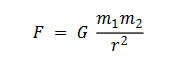Gravitational Force is independent of the medium. The gravitational force inside a body is zero.

Gravitational Constant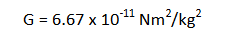Acceleration due to Gravity

Variation with Height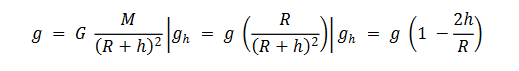Variation with Depth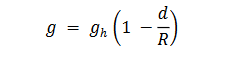for every percentage decrease in radius, with mass constant; there is 2% increase in acceleration due to gravity.

Variation with Latitude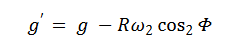Relation between Variation of height and depth

If acceleration due to gravity is same at a certain height and depth;

then d = 2h

Gravitational Field Intensity

Gravitational field is the field of attraction around a mass. Gravitational Field Intensity is the amount of Force experienced by an object of unit mass in a gravitational Field.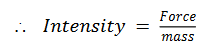Gravitational Potential Energy

Gravitational Potential Energy is the work done in bringing a charge of unit mass from infinity to a point against the gravitational field.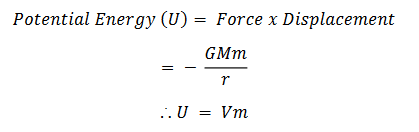Gravitational Potential

Gravitational potential is the amount of work done per unit mass in a gravitational field.

Gravitational Potential is always directed opposite to Gravitational Field.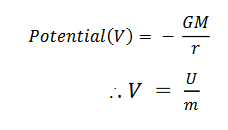Cases of Potential:

1.Due to point mass: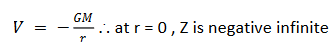The graph will be negative, curved and parallel to V and r axes.

2.Inside a Solid Sphere: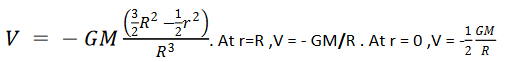The graph is negative, parabolic.

3.Outside a Solid Sphere: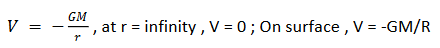4.Due to a Spherical Shell: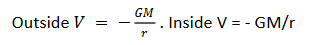5.On the Axis of a Ring: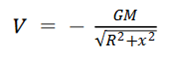Kepler's Laws

Law of Orbits Kepler's Law of Orbits states that all planets revolve around the sun in elliptical orbits with sun as the focus.

Law of Areas

Kepler's Law of Areas states that a planet sweeps equal Areas in equal intervals of time around the sun.

Law of Periods

Kepler's Law of Periods states that the square of Time Period is directly proportional to the cube of Distance.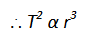Critical Velocity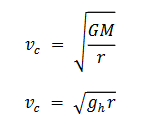Time Period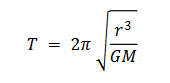Binding Energy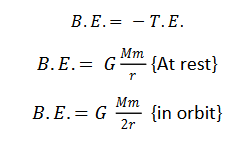Escape Velocity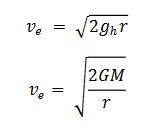The escape velocity remains independent of angle of projection. Escape Velocity for an object on earth is 11.2 km/s.

GRAVITATIONAL POTENTIAL & FIELD DUE TO VARIOUS OBJECTS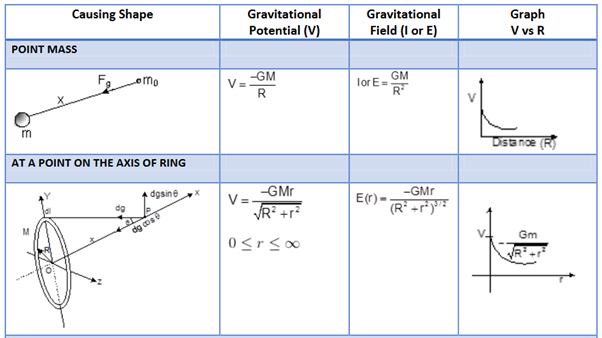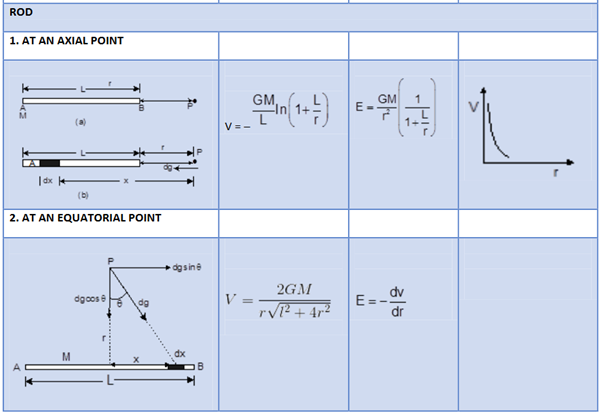Post By : Ravindra Yadav 07 Nov, 2019 3229 views Physics